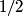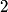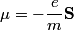## Solution to 1986 Problem 27

 (A) If the electron did not have spin, there would only be one electron state for each quantum number triplet (n,l,m) instead of two. So, the shells and subshells would be filled a lot quicker and, for example, boron would be a Noble gas. Therefore, this answer is not correct. (B) The heat capacity of a metal, as calculated in the free electron Fermi gas model, for example, relies heavily on the fact that electrons have a spin of$1/2$, which causes them to obey Fermi-Dirac statistics and also introduced a degeneracy factor of$2$. Therefore, this answer is not correct. (C)The anomalous Zeeman effect arises because of the interaction between an electron's spin and an external magnetic field. There, this answer is not correct. (D) The deflection of a moving electron by a uniform magnetic field follows simply from the Lorentz force law. Therefore, this answer is correct. (E) The fine-structure correction to the Bohr energies is due in part to the spin-orbit correction and in part to the relativistic correction. The spin-orbit correction refers to the correction caused by the interaction of the electron's intrinsic magnetic moment\begin{align*}\mu = -\frac{e}{m}\mathbf{S}\end{align*}and the magnetic field that the proton creates in the electron's frame of reference. Therefore, this answer is not correct.